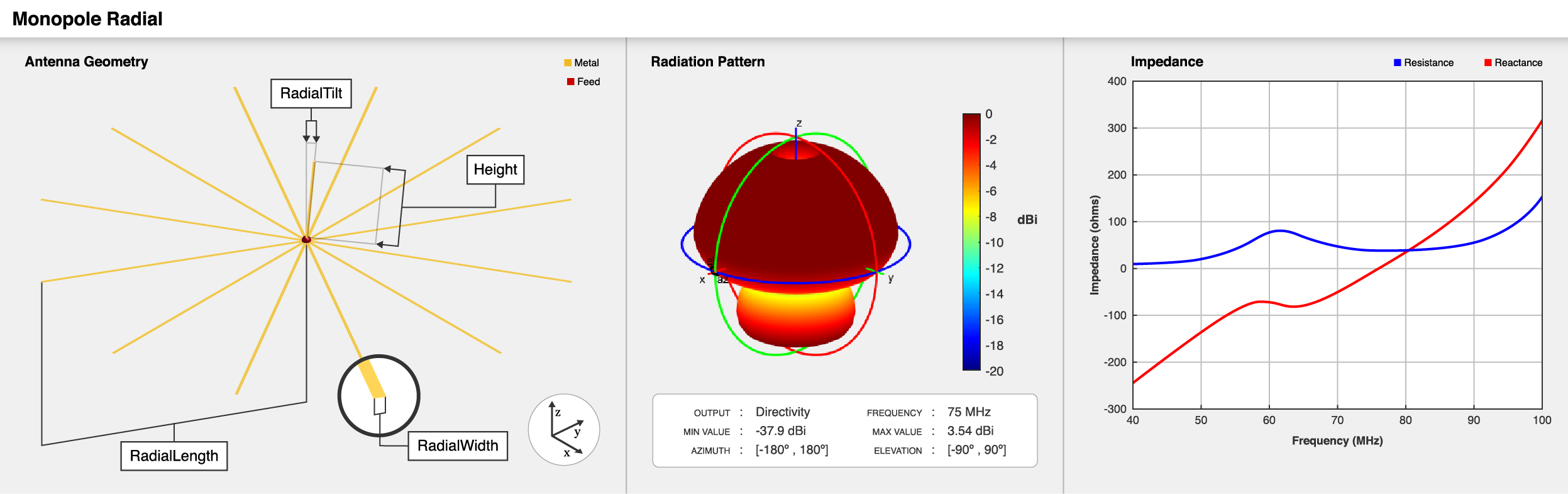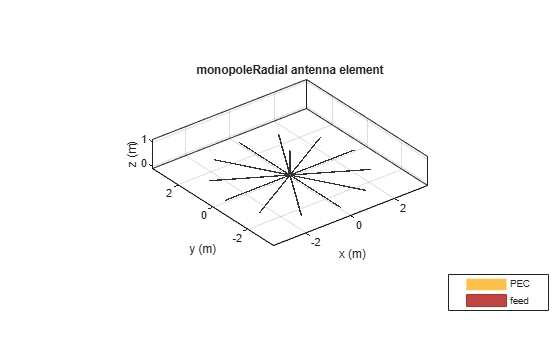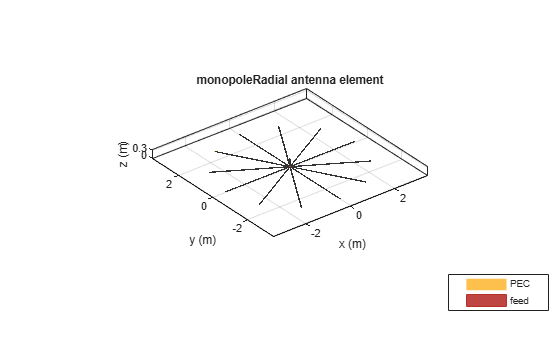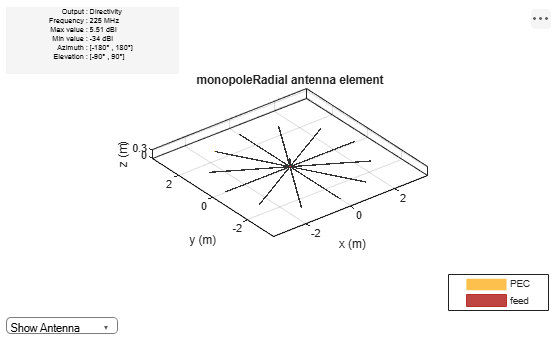Create monopole antenna mounted on radial ground plane

## Description

The `monopoleRadial` antenna object creates a monopole antenna mounted on a radial ground plane. The monopole radial antenna is a variant of the monopole antenna, where the antenna is mounted on radials as versus a rectangular ground plane. These antennas are commonly used in airborne and ground-based radio communications.## Creation

### Syntax

``mpr = monopoleRadial``
``mpr = monopoleRadial(Name,Value)``

### Description

example

``` `mpr = monopoleRadial` creates a quarter wavelength monopole antenna with a radial ground plane. The default antenna object is center-fed with the feed point located at the origin on the X-Y plane. The default antenna object resonates at 75 MHz.```

example

``` `mpr = monopoleRadial(Name,Value)` sets Properties using name-value pairs. For example, `monopoleRadial('Height',2.2)` creates a monopole antenna mounted on a radial ground plane with height of 2.2 meters. You can specify multiple name-value pairs. Enclose each property name in quotes. Properties not specified retain their default values.```

## Properties

expand all

Monopole height, specified as a positive scalar in meter.

Example: `'Height',3`

Data Types: `double`

Monopole width, specified as a positive scalar in meters.

Note

Monopole width should be less than `'Height'`/4 and greater than `'Height'`/1001. For more information, see .

Example: `'Width',0.05`

Data Types: `double`

Number of radials, specified as a positive scalar.

Example: `'NumRadials',14`

Data Types: `double`

Width of each radial in the monopole radial antenna, specified as a positive scalar in meters.

Example: `'RadialWidth',0.05`

Data Types: `double`

Length of each radial in the monopole radial antenna, specified as a positive scalar in meters.

Example: `'RadialLength',3.13`

Data Types: `double`

Tilt angle of radials with respect to the ground plane, specified as a scalar in degree. Radials are tilted along the X-Y plane in negative Z direction.

Example: `'RadialTilt',10`

Data Types: `double`

Type of the metal used as a conductor, specified as a metal material object. You can choose any metal from the `MetalCatalog` or specify a metal of your choice. For more information, see `metal`. For more information on metal conductor meshing, see Meshing.

Example: `m = metal('Copper'); 'Conductor',m`

Example: `m = metal('Copper'); ant.Conductor = m`

Lumped elements added to the antenna feed, specified as a `lumpedElement` object. You can add a load anywhere on the surface of the antenna. By default, the load is at the feed. For more information, see `lumpedElement`.

Example: `'Load',lumpedelement`, where `lumpedelement` is the load added to the antenna feed.

Example: `ant.Load = lumpedElement('Impedance',75)`

Tilt angle of the antenna, specified as a scalar or vector with each element unit in degrees. For more information, see Rotate Antennas and Arrays.

Example: `'Tilt',90`

Example: `ant.Tilt = 90`

Example: `'Tilt',[90 90]`,`'TiltAxis',[0 1 0;0 1 1]` tilts the antenna at 90 degrees about the two axes defined by the vectors.

Note

The `wireStack` antenna object only accepts the dot method to change its properties.

Data Types: `double`

Tilt axis of the antenna, specified as:

• Three-element vector of Cartesian coordinates in meters. In this case, each coordinate in the vector starts at the origin and lies along the specified points on the X-, Y-, and Z-axes.

• Two points in space, each specified as three-element vectors of Cartesian coordinates. In this case, the antenna rotates around the line joining the two points in space.

• A string input describing simple rotations around one of the principal axes, 'X', 'Y', or 'Z'.

Example: `'TiltAxis',[0 1 0]`

Example: `'TiltAxis',[0 0 0;0 1 0]`

Example: `ant.TiltAxis = 'Z'`

Note

The `wireStack` antenna object only accepts the dot method to change its properties.

Data Types: `double`

## Object Functions

 `show` Display antenna or array structure; display shape as filled patch `impedance` Input impedance of antenna; scan impedance of array `sparameters` Calculate S-parameter for antenna and antenna array objects `returnLoss` Return loss of antenna; scan return loss of array `vswr` Voltage standing wave ratio of antenna `optimize` Optimize antenna or array using SADEA optimizer `pattern` Radiation pattern and phase of antenna or array; Embedded pattern of antenna element in array `patternAzimuth` Azimuth pattern of antenna or array `patternElevation` Elevation pattern of antenna or array `axialRatio` Axial ratio of antenna `beamwidth` Beamwidth of antenna `current` Current distribution on metal or dielectric antenna or array surface `charge` Charge distribution on metal or dielectric antenna or array surface `efficiency` Radiation efficiency of antenna `EHfields` Electric and magnetic fields of antennas; Embedded electric and magnetic fields of antenna element in arrays `mesh` Mesh properties of metal or dielectric antenna or array structure `design` Design prototype antenna or arrays for resonance around specified frequency `rcs` Calculate and plot radar cross section (RCS) of platform, antenna, or array

## Examples

collapse all

Create and view a monopole antenna mounted on radial ground plane with default properites.

```ant = monopoleRadial; show(ant)```Create and view a monopole antenna on a radial ground plane with the width of 0.0067 meters and the height of 0.33331 meters.

``` m = monopoleRadial('Width',0.0067,'Height',0.3331); show(m)```Plot the radiation pattern of the antenna at a frequency of 225 MHz.

`pattern(m,225e6)`Balanis, Constantine A. Antenna Theory: Analysis and Design. 3rd ed. Hoboken, NJ: John Wiley, 2005.

 Volakis, John. Antenna Engineering Handbook, 4th Ed. New York: Mcgraw-Hill, 2007.